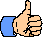# Attribute for `<FORM ...>``NAME = "text string"`

Usage Recommendationuse it

`NAME` gives a name to the form. This is most useful in scripting, where you frequently need to refer to the form in order to refer to the element within the form. For example, this reduced version of our geometry calculating script uses `NAME` to refer to the form which holds the radius field:

```<SCRIPT>
<!--
function Circle_calc_ii()
{
{
document.MyCircleForm.Circle_circumference.value =  2 * Math.PI * CircleRadius ;
document.MyCircleForm.Circle_area.value =  Math.PI * Math.pow(CircleRadius, 2) ;
}
else
{
document.MyCircleForm.Circle_circumference.value =  "";
document.MyCircleForm.Circle_area.value =  "";
}
}
// -->
</SCRIPT>
<FORM NAME="MyCircleForm">
<TR>
<TD><INPUT TYPE=BUTTON OnClick="Circle_calc_ii(this.form);" VALUE="calculate"></TD>
<TD ALIGN=RIGHT BGCOLOR="#AACCFF">
<NOBR>circumference: <INPUT NAME="Circle_circumference" SIZE=9></NOBR><BR>
<NOBR>area: <INPUT NAME="Circle_area" SIZE=9></NOBR></TD>
</TR>
</TABLE>
</FORM>
```

It is possible to refer to the form without using `NAME`, and sometimes it makes for less work. To give the above example we needed to create the whole script all over again. Instead, we could use the original CircleAreaCalc function at the top of this page, which allows us to pass in the form object as an argument (using `this.form`), obviating the need for `NAME`:

```<FORM><!-- note there is no NAME atttibute -->
<!-- circumference and radius of a circle -->
<TR>
<TD><INPUT TYPE=BUTTON OnClick="Circle_calc(this.form);" VALUE="calculate"></TD>
<TD ALIGN=RIGHT BGCOLOR="#AACCFF">
<NOBR>circumference: <INPUT NAME="Circle_circumference" SIZE=9></NOBR><BR>
<NOBR>area: <INPUT NAME="Circle_area" SIZE=9></NOBR></TD>
</TR>
</TABLE>
</FORM>
```

which gives us

Another much worse way to refer to the form without giving it a name is to use the form's index in the forms array. If our form were the first form on this web page, we could refer to it like this:

```var CircleRadius = parseFloat(document.forms.Circle_radius.value);
```

That would require keeping track of how many forms down on the page it is, and on a page like this that would be way too much trouble.